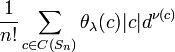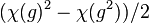# Formula for calculating effect of Schur functor on character

## Statement

Suppose$G$ is a group,$K$ is a field (which we will assume for simplicity to have characteristic zero) and$\varphi:G \to GL(d,K)$ is a linear representation of$G$ over$K$ with character$\chi$. Suppose$\lambda$ is a partition of a positive integer$n$ and denote by$\mathbb{S}_\lambda$ the Schur functor corresponding to$\lambda$. We can consider a new representation$\mathbb{S}_\lambda(\varphi)$ and we denote its character by$\mathbb{S}_\lambda(\chi)$. Our goal is to provide an explicit description of$\mathbb{S}_\lambda(\chi)$.

### Explicit description of degree of representation

The new degree is a polynomial in the old degree. The degree of the polynomial (not to be confused with degrees of representations) equals$n$. Explicitly, the polynomial is defined as follows. Denote by$C(S_n)$ the set of conjugacy classes of the symmetric group$S_n$. Let$\nu:C(S_n) \to \mathbb{N}$ be the function that outputs the number of cycles of a given conjugacy class. Denote by$\theta_\lambda$ the character of the irreducible representation of$S_n$ corresponding to the partition$\lambda$ of$n$. Then, the polynomial is:$\frac{1}{n!} \sum_{c \in C(S_n)} \theta_\lambda(c) |c| d^{\nu(c)}$

### Explicit description of character of representation

The character of the representation is given as follows. Denote by$C(S_n)$ the set of conjugacy classes of the symmetric group$S_n$. Let$\nu:C(S_n) \to \mathbb{N}$ be the function that outputs the number of cycles of a given conjugacy class. Denote by$\theta_\lambda$ the character of the irreducible representation of$S_n$ corresponding to the partition$\lambda$ of$n$. Then, the character is:$\mathbb{S}_\lambda(\chi)(g) = \frac{1}{n!} \sum_{c \in C(S_n)} \theta_\lambda(c) |c| \left(\prod_{\mbox{parts in the cycle type of } c} \chi(g^{\mbox{size of the part}})\right)$

For instance, for a conjugacy class in$S_9$ with cycle type$3 + 3 + 2 + 1$, the product on the inside reads$\chi(g^3)\chi(g^3)\chi(g^2)\chi(g)$.

## Description for small Schur functors

Size of set being partitioned Partition for Schur functor Name of functor Formula for computing degree if original representation has degree$d$ Formula for computing character of the representation obtained after applying this functor in terms of the original character$\chi$, on an element$g$ Explanation for character formula
1 1 identity functor$d$$\chi(g)$$n = 1$, so the only summand corresponds to the partition 1 of 1, and is$\frac{1}{1!} (1)(1)\chi(g^1) = \chi(g)$.
2 2 symmetric square$d(d + 1)/2$$(\chi(g)^2 + \chi(g^2))/2$$n = 2$ and$\lambda$ is the partition 2, so the summation is carried out over the partitions of 2. The representation$\theta_\lambda$ is the trivial representation. The two parts are:
partition 1 + 1 of 2, corresponding to conjugacy class of identity element: the summand is$\frac{1}{2!}(1)(1)\chi(g)\chi(g) = (\chi(g))^2/2$.
partition 2 of 2, corresponding to conjugacy class of non-identity element: the summand is$\frac{1}{2!}(1)(1)\chi(g^2) = \chi(g^2)/2$.
2 1 + 1 exterior square or alternating square$d(d - 1)/2$$(\chi(g)^2 - \chi(g^2))/2$
3 3 symmetric cube$d(d + 1)(d + 2)/6$$(\chi(g)^3 + 3\chi(g^2)\chi(g) + 2\chi(g^3))/6$
3 2 + 1  ?$d(d + 1)(d - 1)/3$$(\chi(g)^3 - \chi(g^3))/3$
3 1 + 1 + 1 exterior cube$d(d - 1)(d - 2)/6$$(\chi(g)^3 - 3\chi(g^2)\chi(g) + 2\chi(g^3))/6$